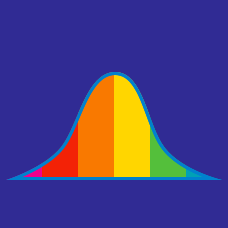Probability

# Variance - Properties

The probability distribution of a random variable $X$ is

$\begin{array}{c}&P(X = 1) = 0.1 &P(X = 2) = 0.2 &P( X = 3) = 0.3 &P( X = 4) = 0.4. \end{array}$

If $Y = 9X+14,$ what is the variance of $Y?$

A random variable $X$ has $E(X)=3$ and $V(X)=9.$ If $\begin{array}{c}&Y=aX+b, &E(Y)= 6, &V(Y) = 9,\end{array}$ what are $a(>0)$ and $b?$

If $V(X) = 4$, what is $V(2X + 7 )?$

If $V(X) = 3$ and $E(X^2) = 10,$ what is $(E(X))^2 ?$

If $V(X) = 5$, what is $V(2X + 4 )?$

×